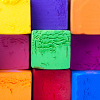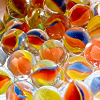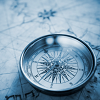# Geometry and Measure

This is part of our Secondary Curriculum collection of favourite rich tasks arranged by topic.### Angles, Polygons and Geometrical Proof (age 11-14)

Working on these problems will help your students develop a better understanding of angles, polygons and proof.### Angles, Polygons and Geometrical Proof (age 14-16)

Working on these problems will help your students develop a better understanding of angles, polygons and proof.### Construction### 3D Shapes### Perimeter, Area and Volume (age 11-14)

Working on these problems will help your students develop a better understanding of perimeter, area and volume.### Perimeter, Area and Volume (age 14-16)

Working on these problems will help your students develop a better understanding of perimeter, area and volume.### Transformations